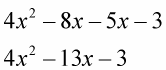##### Self-Publishing For DummiesWhen performing distribution, be aware of the sign being distributed and how that sign affects each term. Distributing a positive sign makes no difference in the signs of the terms — the signs stay the same. When distributing a negative sign, each term has a change of sign from negative to positive or from positive to negative.

Check out these examples to see the distribution of positive and negative numbers in action, and notice how the terms do — or don't — change.

• Distribute a positive number.

Example 1: +(4x + 2y – 3z + 7) is the same as multiplying through by +1, so

+1(4x + 2y – 3z + 7)

+ 1(4x) + 1(2y) + 1(–3z) + 1(7)

4x + 2y – 3z + 7

The signs don’t change.

Example 2: +3(4x + 2y – 3z + 7)

+3(4x) + 3(2y) + 3(–3z) + 3(7)

12x + 6y – 9z + 21

The signs don’t change.

• Distribute a negative number.

Example 1: –(4x + 2y – 3z + 7) is the same as multiplying through by −1, so

−1(4x + 2y – 3z + 7)

–1(4x) –1(2y) –1(–3z) –1(7)

–4x – 2y + 3z – 7

Each term changes to the opposite sign.

Example 2: 4x(x – 2) – (5x + 3)

4x(x – 2) = 4x(x) – 4x(2)

–(5x + 3) = –(+5x) – (+3)

4x(x) – 4x(2) – (+5x) – (+3)The tricky part is to make sure that you distribute the negative sign over the 5x and the 3.

One mistake to avoid when you’re distributing a negative sign is not distributing over all the terms. This is especially the case when the process is hidden. Hidden means that a negative sign may not be in front of the whole expression, where it stands out. It can be between terms, showing a subtraction and not being recognized for what it is. Don’t let the negative signs ambush you.